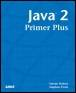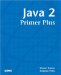# ExercisesJava™ 2 Primer PlusBy Steven Haines, Steve Potts Table of Contents Chapter 4.  Flow Control

 1: Write a program that displays the temperatures from 0 degrees Celsius to 100 degrees Celsius and its Fahrenheit equivalent. Note that the conversion from Celsius to Fahrenheit uses the following formula: ` F = C * 9/5 + 32; ` 2: Write a program that displays all prime numbers from 1 to 100. Note that a prime number is a number that is only divisible by itself and 1. For example the number 7 is prime, but the number 6 is not: ` 6 = 6*1 and 2*3 `

 TopJava 2 Primer Plus
ISBN: 0672324156
EAN: 2147483647
Year: 2001
Pages: 332

Similar book on Amazon